# KNN Model Complexity

• Difficulty Level : Medium
• Last Updated : 05 Sep, 2020

KNN is a machine learning algorithm which is used for both classification (using KNearestClassifier) and Regression (using KNearestRegressor) problems.In KNN algorithm K is the Hyperparameter. Choosing the right value of K matters. A machine learning model is said to have high model complexity if the built model is having low Bias and High Variance.

We know that,

1. High Bias and Low Variance = Under-fitting model.
2. Low Bias and High Variance = Over-fitting model. [Indicated highly complex model ].
3. Low Bias and Low Variance = Best fitting model. [This is preferred ].
4. High training accuracy and Low test accuracy ( out of sample accuracy ) = High Variance = Over-fitting model = More model complexity.
5. Low training accuracy and Low test accuracy ( out of sample accuracy ) = High Bias = Under-fitting model.

Code: To understand how K value in KNN algorithm affects the model complexity.

 `# This code may not run on GFG ide ` `# As required modules are not found. ` `  `  `# Import required modules ` `import` `matplotlib.pyplot as plt ` `from` `sklearn.datasets ``import` `make_regression ` `from` `sklearn.neighbors ``import` `KNeighborsRegressor ` `from` `sklearn.model_selection ``import` `train_test_split ` `import` `numpy as np ` `  `  `# Synthetically Create Data Set ` `plt.figure() ` `plt.title(``'SIMPLE-LINEAR-REGRESSION'``) ` `x, y ``=` `make_regression( ` `    ``n_samples ``=` `100``, n_features ``=` `1``,  ` `    ``n_informative ``=` `1``, noise ``=` `15``, random_state ``=` `3``) ` `plt.scatter(x, y, color ``=``'red'``, marker ``=``'o'``, s ``=` `30``) ` `  `  `# Train the model. ` `knn ``=` `KNeighborsRegressor(n_neighbors ``=` `7``) ` `x_train, x_test, y_train, y_test ``=` `train_test_split( ` `    ``x, y, test_size ``=` `0.2``, random_state ``=` `0``) ` `knn.fit(x_train, y_train) ` `predict ``=` `knn.predict(x_test) ` `print``(``'Test Accuracy:'``, knn.score(x_test, y_test)) ` `print``(``'Training Accuracy:'``, knn.score(x_train, y_train)) ` `  `  `# Plot The Output ` `x_new ``=` `np.linspace(``-``3``, ``2``, ``100``).reshape(``100``, ``1``) ` `predict_new ``=` `knn.predict(x_new) ` `plt.plot( ` `    ``x_new, predict_new, color ``=``'blue'``,  ` `    ``label ``=``"K = 7"``) ` `plt.scatter(x_train, y_train, color ``=``'red'` `) ` `plt.scatter(x_test, predict, marker ``=``'^'``, s ``=` `90``) ` `plt.legend() `

Output: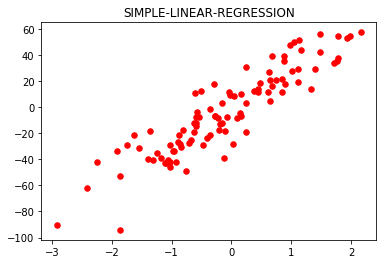```Test Accuracy: 0.6465919540035108
Training Accuracy: 0.8687977824212627
```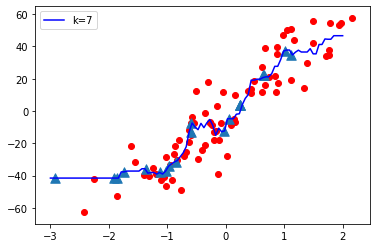Now let’s vary the value of K (Hyperparameter) from Low to High and observe the model complexity
K = 1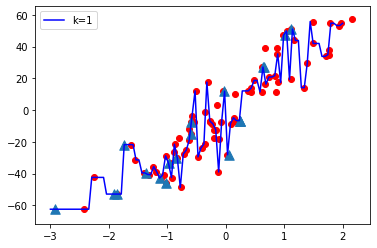K = 10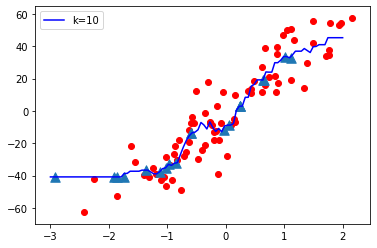K = 20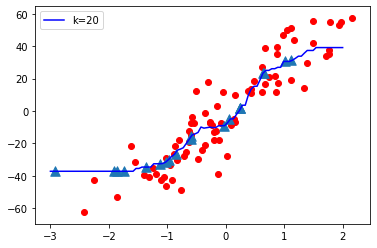K = 50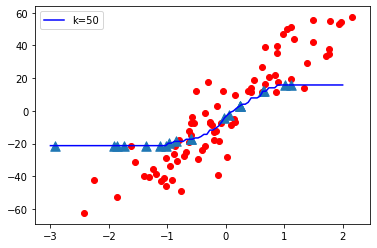K = 70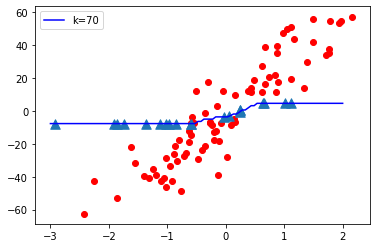Observations:

• When K value is small i.e. K=1, The model complexity is high ( Over-fitting or High Variance).
• When K value is very large i.e. K=70, The model complexity decreases ( Under-fitting or High Bias ).

Conclusion:
As K value becomes small model complexity increases and as K value becomes large the model complexity decreases.

Code: Let’s consider the below plot

 `# This code may not run on GFG ` `# As required modules are not found. ` ` `  `# To plot test accuracy and train accuracy Vs K value. ` `p ``=` `list``(``range``(``1``, ``31``)) ` `lst_test ``=``[] ` `lst_train ``=``[] ` `for` `i ``in` `p: ` `    ``knn ``=` `KNeighborsRegressor(n_neighbors ``=` `i) ` `    ``knn.fit(x_train, y_train) ` `    ``z ``=` `knn.score(x_test, y_test) ` `    ``t ``=` `knn.score(x_train, y_train) ` `    ``lst_test.append(z) ` `    ``lst_train.append(t) ` `     `  `plt.plot(p, lst_test, color ``=``'red'``, label ``=``'Test Accuracy'``) ` `plt.plot(p, lst_train, color ``=``'b'``, label ``=``'Train Accuracy'``) ` `plt.xlabel(``'K VALUES --->'``) ` `plt.title(``'FINDING BEST VALUE FOR K'``) ` `plt.legend() `

Output: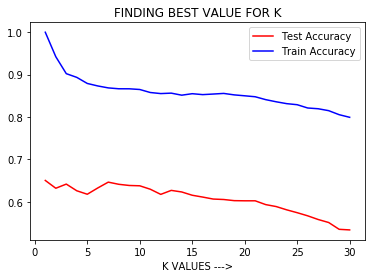Observation:
From the above graph, we can conclude that when K is small i.e. K=1, Training Accuracy is High but Test Accuracy is Low which means the model is over-fitting ( High Variance or High Model Complexity). When the value of K is large i.e. K=50, Training Accuracy is Low as well as Test Accuracy is Low which means the model is under-fitting ( High Bias or Low Model Complexity ).

So Hyperparameter tuning is necessary i.e. to select the best value of K in KNN algorithm for which the model has Low Bias and Low Variance and results in a good model with high out of sample accuracy.

We can use GridSearchCV or RandomSearchCv to find the best value of hyper parameter K.

My Personal Notes arrow_drop_up
Recommended Articles
Page :